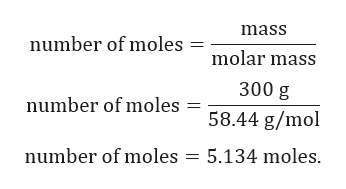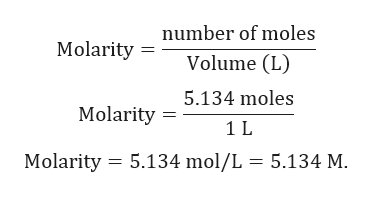Question
61 views

What is the molarity of a 30% solution of NaCl?

check_circle

Step 1

Given:

For NaCl, a 30% solution would be 30 g of NaCl in 100 mL of Solution, so multiplying by 10 would give 300 g in 1 L of solution.

Molar mass of NaCl = 58.44 g/mol.

Step 2

Calculation for number of moles of NaCl:help_outlineImage Transcriptionclosemass number of moles molar mass 300 g number of moles 58.44 g/mol number of moles 5.134 moles fullscreen
Step 3

Calculation for molari...help_outlineImage Transcriptionclosenumber of moles Molarity Volume (L) 5.134 moles Molarity 1 L Molarity 5.134 mol/L 5.134 M. fullscreen

### Want to see the full answer?

See Solution

#### Want to see this answer and more?

Solutions are written by subject experts who are available 24/7. Questions are typically answered within 1 hour.*

See Solution
*Response times may vary by subject and question.
Tagged in

### Solutions